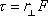tau = r-perp F

Torque = moment arm times force

This is an expression for calculating the magnitude of the torque resulting from a force F. It states that the magnitude of the torque due to force F, with respect to a specified axis of rotation, is equal to the moment arm, with respect to that same axis of rotation, times the magnitude of the force.

The moment arm is the distance from the axis of rotation to the line of action of the force measured along a line that is perpendicular to the line of action of the force. In other words, it is the shortest distance from the axis of rotation to the line of action of the force.